Discovering statistics using IBM SPSS statistics by Andy Field, fifth edition – Summary chapter 1

The research process generally starts with an observation. After the observation, relevant theories are consulted and hypotheses are generated, from which predictions are made. After that, data is collected to test the predictions and finally the data is analysed. The data analysis either supports or does not support the hypothesis. A theory is an explanation or set of principles that is well substantiated by repeated testing and explains a broad phenomenon. A theory should be able to explain all of the data. A hypothesis is a proposed explanation for a fairly narrow phenomenon or set of observations. Hypotheses are theory-driven. Predictions are often used to move from the conceptual domain to the observable domain to be able to collect evidence. Falsification is the act of disproving a hypothesis or theory. A scientific theory should be falsifiable and explain as much of the data as possible.

DATA
Variables are things that can vary. An independent variable is a variable thought to be the cause of some effect and is usually manipulated, in research. A dependent variable is a variable thought to be affected by changes in an independent variable. The predictor variable is a variable thought to predict an outcome variable (independent variable). The outcome variable is a variable thought to change as a function of changes in a predictor a predictor variable (dependent variable). The difference between dependent variables and outcome variables is that one is about experimental research and the other is applicable to both experimental and correlational research.

The level of measurement is the relationship between what is being measured and the numbers that represent what is being measured. A categorical variable is made up of categories. There are three types of categorical variables:

1. Binary variable
A categorical variable with two options (e.g. ‘yes’ or ‘no’).
2. Nominal variable
A categorical variable with more than two options (e.g. hair colour).
3. Ordinal variables
A categorical variable that has been ordered (e.g. winner and runner-up)

Nominal data can be used when considering frequencies. Ordinal data does not tell us anything about the difference between points on a scale. A continuous variable is a variable that gives us a score for each person and can take on any value. An interval variable is a continuous variable with equal differences between the intervals (e.g. the difference between a ‘9’ and a ‘10’ on a grade). Ratio variables are continuous variables in which the ratio has meaning (e.g. a rating of ‘4’ is twice as good as a rating of ‘2’). Ratio variables require a meaningful zero point. A discrete variable is a variable that can take on only certain values.

Measurement error is the discrepancy between the numbers we use to represent the thing we’re measuring and the actual value of this thing. Self-report will produce larger measurement error. Validity is whether an instrument measures what it sets out to measure. Reliability is whether an instrument can be interpreted consistently across different situations. One way of testing reliability is using the test-retest reliability: testing and retesting at a later time to see whether the results are the same.

There are different types of validity:

1. Criterion validity
This is whether you can establish that an instrument measures what it claims to measure through comparison to objective criteria.
2. Concurrent validity
This is whether a measurement is similar to an existing measurement of the same thing.
3. Predictive validity
This is whether an instrument can predict observations at a later point in time.
4. Content validity
This is the degree to which an assessment instrument is relevant to, and representative of, the targeted construct it is designed to measure.

RESEARCH DESIGN
In correlational or cross-sectional research observations are made without interfering and in experimental research a variable is manipulated. Ecological validity is the extent to which the conclusions of a research can be generalized to the world. Correlational research can be used if variables are difficult to manipulate or if it is unethical to manipulate variables. A limitation of drawing conclusions based on correlational research is the tertium quid, the risk of a third variable. These third variables are called confounding variables.

There are different research designs for experimental research:

1. Between-groups, between-subjects or independent design
Each group or subject experiences a different manipulation of the independent variable or no manipulation (control group).
2. Within-subject or repeated-measures design
Each subject experiences multiple manipulations of the independent variable or goes through all the different manipulations of the independent variable.

If participants do the same thing twice without changing the manipulation, there will be unsystematic variation, small variation in performance. If the manipulation is different and actually has an effect, then there will be systematic variation. Systematic variation can occur because of a change in manipulation or because other factors differ, (un)related to the manipulation.

Randomization is used to prevent unwanted systematic variation and keep the unsystematic variation to a minimum. Practice effects may occur in within-subjects design, because participants become familiar with the measures used. Boredom effects may occur in within-subjects design because they are tired or bored from having completed the first condition. This can lead to systematic variation if counterbalancing is not used, the order in which a participant participates in a condition.

Many naturally occurring things have the shape of a normal distribution (e.g. height). A distribution can deviate from normal by a lack of symmetry, called skew or by pointiness, called kurtosis. A skewed distribution can be positively skewed or negatively skewed. The name is determined by the direction of the tail. Kurtosis refers to the degree to which scores cluster at the ends of the distribution. Positive kurtosis (leptokurtic distribution) has many scores in the tail and is very pointy. Negative kurtosis (platykurtic distribution) is thin in the tails and tends to be flatter than normal.

The mode is the score that occurs most frequently in the data set. A data set with two modes is bimodal and a data set with more than two modes is multimodal. The median is the middle number. The median is relatively unaffected by extreme scores. The mean is the average. It uses the following formula: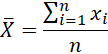It means adding up all the scores then dividing by the total number of scores. Quantiles are values that split a data set into equal portions. Percentiles split the data into 100 equal parts and noniles split the data into nine equal parts. The interquartile range is the difference between quartile three and quartile one. The difference between each score and the mean is the deviance and uses the following formula: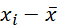It is a score minus the mean. The total deviance is the sum of all the deviances and uses the following formula: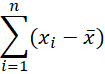The total deviance is always zero. The sum of squared errors (SS) sums up all the squared deviation. It is an indicator of the total deviance of scores from the mean and uses the following formula: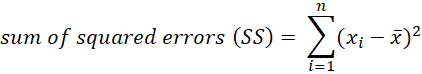One problem of the sum of squares is that it cannot be used to compare two samples of different sample sizes. The variance is the average dispersion and this can be used to compare between two samples with different sample sizes. It is SS divided by sample size minus one and uses the following formula: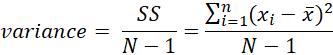It uses units squared, which makes it difficult to see any practicality in it, which is why the standard deviation is used. This is the square root of the variance and uses the following formula: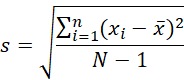A small standard deviation indicates that the data points are close to the mean. Probability density functions are mathematical formulae that specify idealized versions of distributions. In order to calculate probabilities of things happening using standard deviations the z-score can be used. It shows us how many standard deviation an observation falls from the mean, which can be linked to probabilities using the z-table. It uses the following formula: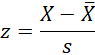There are some guidelines for reporting data:

1. Choose a mode of presentation that optimizes the understanding of the data
2. If you present three or fewer numbers then try using a sentence
3. If you need to present between 4 and 20 numbers consider a table
4. If you need to present more than 20 numbers use a graph

Selected Categories
Connect & Continue
WorldSupporter Resources

#Discovering statistics using IBM SPSS statistics by Andy Field, fifth edition – Book summary

This bundle contains the chapters of the book "Discovering statistics using IBM SPSS statistics by Andy Field, fifth edition". It includes the following chapters:

- 1, 2, 3, 5, 6, 7, 8, 9, 10, 11, 12, 13, 14, 15, 16, 17, 18.

This Summary is part of the following bundle(s)

This question is for testing whether or not you are a human visitor and to prevent automated spam submissions.Join World Supporter
Join World Supporter

## Why create an account?

• Once you are logged in, you can:
• Save pages to your favorites
• Give feedback or share contributions
• participate in discussions
• share your own contributions through the 7 WorldSupporter tools
Content